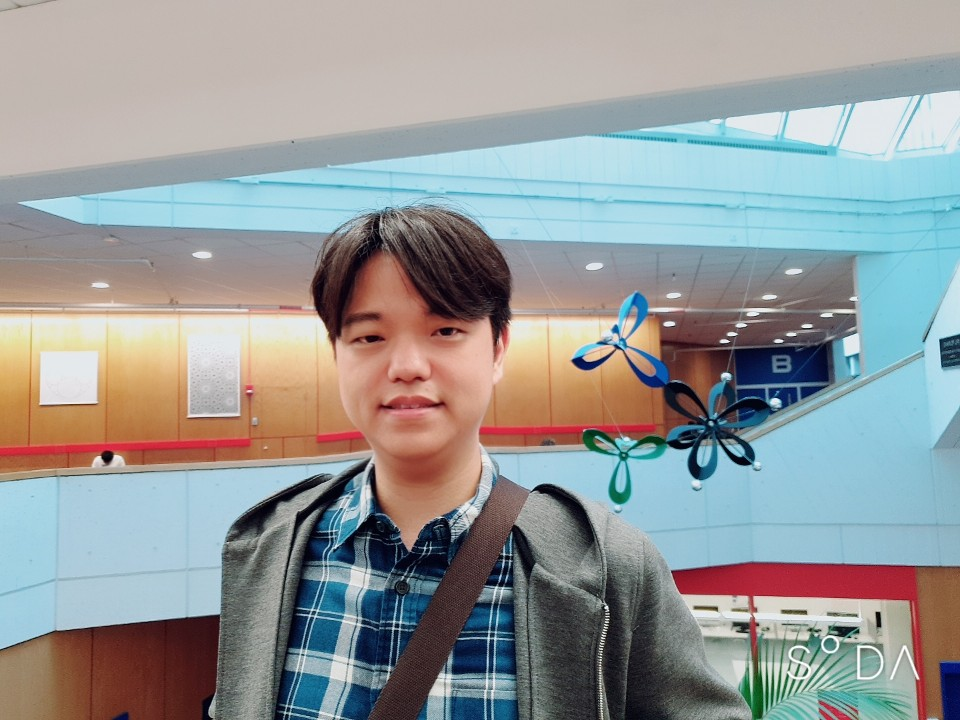# Changho HanPostal address: Pure Mathematics, University of Waterloo, 200 University Avenue West Waterloo, Ontario, Canada N2L 3G1
Email address: changho (dot) han (at) uwaterloo (dot) ca
Office: MC 6457

About me: I am a Postdoctoral Fellow at the University of Waterloo. My research is primarily in algebraic geometry. In particular, I am mainly interested in geometric and arithmetic properties of moduli spaces, and also interested in enumerative problems. See my research statement for more details on my mathematical interests.

I got PhD degree in Mathematics at Harvard University. My graduate advisor is Joe Harris.

I was a Limited-Term Assistant Professor at the University of Georgia.

Here is my CV.

## Teaching

I have taught the following at the University of Waterloo:

• Amath/Pmath 331, as an instructor (Applied Real Analysis), Fall 2023

• Amath/Pmath 332, as an instructor (Applied Complex Analysis), Winter 2023

• Math 127, as an instructor (Calculus 1 for the Sciences), Fall 2022

I have taught the following at the University of Georgia:

• Math 2700, as an instructor (Elementary Differential Equations), Spring 2022

• Math 2250, as an instructor (Calculus I), Fall 2021

• Math 2700, as an instructor (Elementary Differential Equations), Spring 2021

• Math 2250, as an instructor (Calculus I), Fall 2020

• Math 2250, as an instructor (Calculus I), Spring 2020

• Math 1113, as an instructor (precalculus), Fall 2019

I have taught the following courses at Harvard:

• Math 1b, as a coaching fellow (integration, series and differential equations) and as a teaching fellow, Spring 2019

• Math 1b, as a teaching fellow (integration, series and differential equations), Fall 2017

• Math 21b, as a teaching fellow (linear algebra and differential equations), Spring 2017

• Math 258Y, as a course assistant (graduate topics course on degenerations in algebraic geometry), Fall 2016

• Math 1b, as a teaching fellow (integration, series and differential equations), Fall 2015